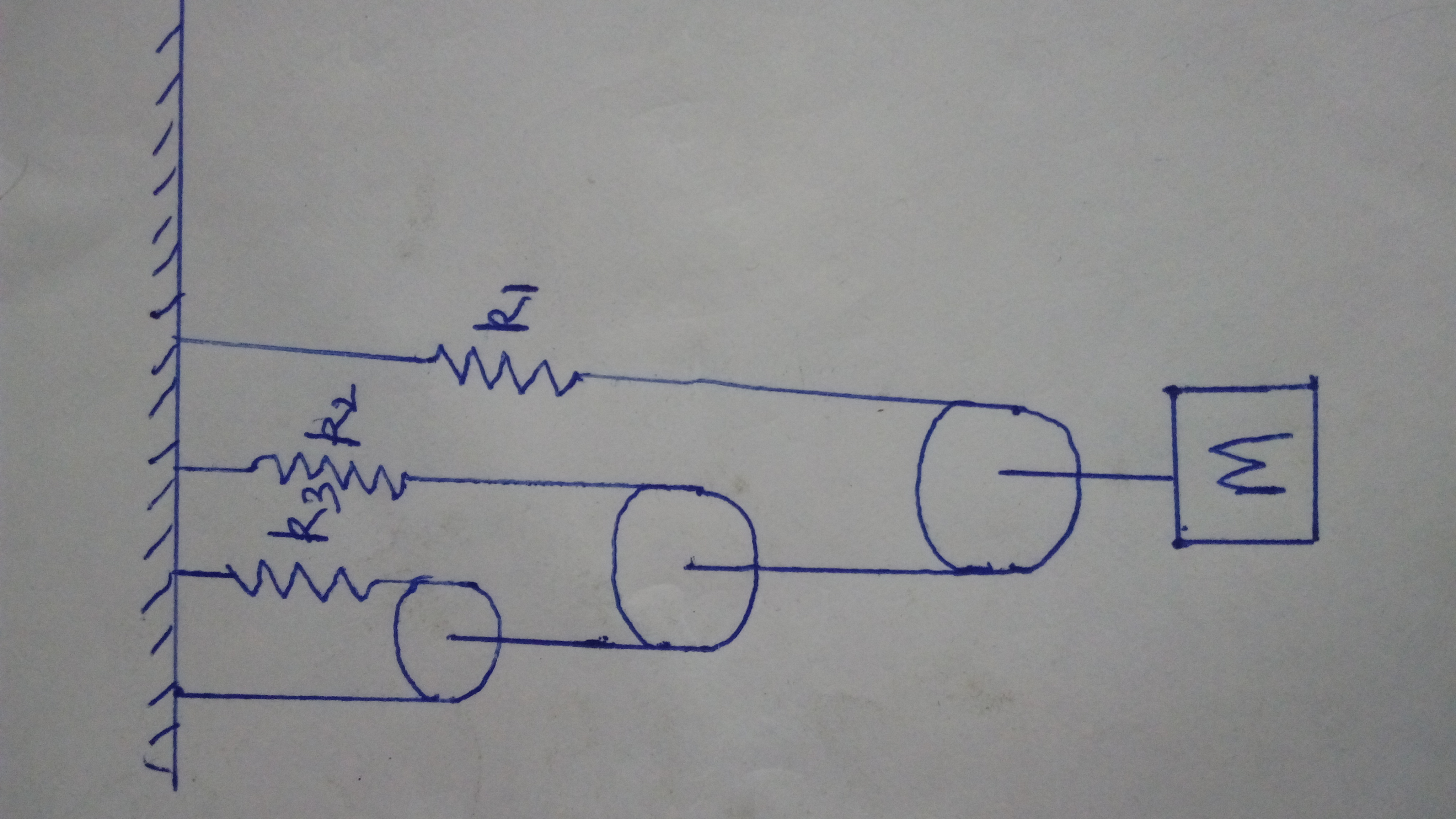# Three pulley systemThe pulley, strings and springs are light.I just wanted to confirm my answer (although I know I am wrong) that

$\Large{\omega^2=\frac{4}{M \left(\frac{1}{k_{1}}+\frac{2}{k_{2}}+\frac{4}{k_{3}}\right)}}$Note by Tanishq Varshney
5 years, 7 months ago

This discussion board is a place to discuss our Daily Challenges and the math and science related to those challenges. Explanations are more than just a solution — they should explain the steps and thinking strategies that you used to obtain the solution. Comments should further the discussion of math and science.

When posting on Brilliant:

• Use the emojis to react to an explanation, whether you're congratulating a job well done , or just really confused .
• Ask specific questions about the challenge or the steps in somebody's explanation. Well-posed questions can add a lot to the discussion, but posting "I don't understand!" doesn't help anyone.
• Try to contribute something new to the discussion, whether it is an extension, generalization or other idea related to the challenge.

MarkdownAppears as
*italics* or _italics_ italics
**bold** or __bold__ bold
- bulleted- list
• bulleted
• list
1. numbered2. list
1. numbered
2. list
Note: you must add a full line of space before and after lists for them to show up correctly
paragraph 1paragraph 2

paragraph 1

paragraph 2

[example link](https://brilliant.org)example link
> This is a quote
This is a quote
    # I indented these lines
# 4 spaces, and now they show
# up as a code block.

print "hello world"
# I indented these lines
# 4 spaces, and now they show
# up as a code block.

print "hello world"
MathAppears as
Remember to wrap math in $$ ... $$ or $ ... $ to ensure proper formatting.
2 \times 3 $2 \times 3$
2^{34} $2^{34}$
a_{i-1} $a_{i-1}$
\frac{2}{3} $\frac{2}{3}$
\sqrt{2} $\sqrt{2}$
\sum_{i=1}^3 $\sum_{i=1}^3$
\sin \theta $\sin \theta$
\boxed{123} $\boxed{123}$

Sort by:

- 5 years, 7 months ago

i'm getting $\large\frac{4}{M(\frac{1}{k_{1}}+\frac{1}{4k_{2}}+\frac{1}{16k_3})}$

- 5 years, 7 months ago

Yeah.

- 5 years, 6 months ago

I'm getting $\frac { 8 }{ M(\frac { 16 }{ { k }_{ 1 } } +\frac { 4 }{ { k }_{ 2 } } +\frac { 1 }{ { k }_{ 3 } } ) }$

- 5 years, 7 months ago

- 5 years, 7 months ago

Take the tension with the string attached to the mass as $8T$. Now, the following strings will have half the tension as the previous one, as you already know. Consider the mass moves by a distance $x$. The spring ${ k }_{ 1 }$ gets stretched by ${ x }_{ 1 }$, ${ k }_{ 2 }$ with ${ x }_{ 2 }$, and ${ k }_{ 3 }$ with ${ x }_{ 3 }$. You can clearly see, that ${ k }_{ 1 }\times { x }_{ 1 }=4T$, ${ k }_{ 2 }\times { x }_{ 2 }=2T$ and ${ k }_{ 3 }\times { x }_{ 3 }=T$ Also by virtual work done: $8x=4{ x }_{ 1 }+2{ x }_{ 2 }+{ x }_{ 3 }$

Put ${ x }_{ 1 }=4a/{ k }_{ 1 }$, ${ x }_{ 2 }=2a/{ k }_{ 2 }$, and ${ x }_{ 3 }=a/{ k }_{ 3 }$. Also, put $x=a/k$ where k is the net inertia factor and $x, k$ will also follow the same relation as the other pairs.

Solving this: ${ \omega }^{ 2 }=\frac { 8 }{ M(\frac { 16 }{ { k }_{ 1 } } +\frac { 4 }{ { k }_{ 2 } } +\frac { 1 }{ { k }_{ 3 } } ) }$

- 5 years, 7 months ago

Are you sure that you didn't exchange the indexes 1 and 3?

- 4 years, 1 month ago